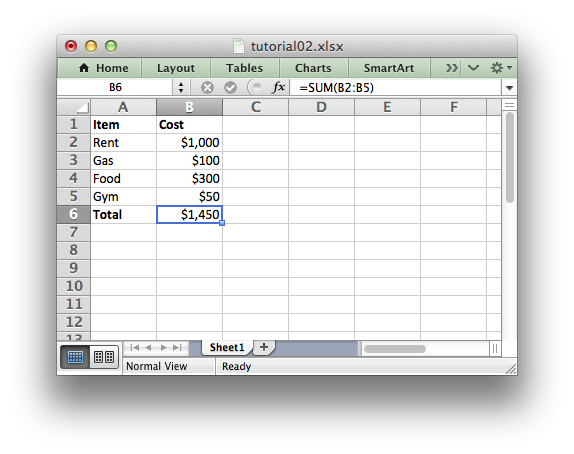# Tutorial 2: Adding formatting to the XLSX File

In the previous section we created a simple spreadsheet using Python and the XlsxWriter module.

This converted the required data into an Excel file but it looked a little bare. In order to make the information clearer we would like to add some simple formatting, like this:The differences here are that we have added Item and Cost column headers in a bold font, we have formatted the currency in the second column and we have made the Total string bold.

To do this we can extend our program as follows:

(The significant changes are shown with a red line.)

``` import xlsxwriter

# Create a workbook and add a worksheet.
workbook = xlsxwriter.Workbook('Expenses02.xlsx')

# Add a bold format to use to highlight cells.

# Add a number format for cells with money.

worksheet.write('A1', 'Item', bold)
worksheet.write('B1', 'Cost', bold)

# Some data we want to write to the worksheet.
expenses = (
['Rent', 1000],
['Gas',   100],
['Food',  300],
['Gym',    50],
)

# Start from the first cell below the headers.
row = 1
col = 0

# Iterate over the data and write it out row by row.
for item, cost in (expenses):
worksheet.write(row, col,     item)
worksheet.write(row, col + 1, cost, money)
row += 1

# Write a total using a formula.
worksheet.write(row, 0, 'Total',       bold)
worksheet.write(row, 1, '=SUM(B2:B5)', money)

workbook.close()
```

The main difference between this and the previous program is that we have added two Format objects that we can use to format cells in the spreadsheet.

Format objects represent all of the formatting properties that can be applied to a cell in Excel such as fonts, number formatting, colors and borders. This is explained in more detail in The Format Class section.

For now we will avoid getting into the details and just use a limited amount of the format functionality to add some simple formatting:

```# Add a bold format to use to highlight cells.

# Add a number format for cells with money.
```

We can then pass these formats as an optional third parameter to the worksheet.`write()` method to format the data in the cell:

```write(row, column, token, [format])
```

Like this:

```worksheet.write(row, 0, 'Total', bold)
```

Which leads us to another new feature in this program. To add the headers in the first row of the worksheet we used `write()` like this:

```worksheet.write('A1', 'Item', bold)
worksheet.write('B1', 'Cost', bold)
```

So, instead of `(row, col)` we used the Excel `'A1'` style notation. See Working with Cell Notation for more details but don’t be too concerned about it for now. It is just a little syntactic sugar to help with laying out worksheets.

In the next section we will look at handling more data types.